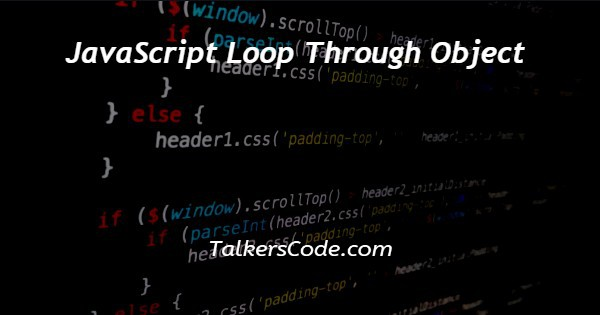# JavaScript Loop Through Object

Last Updated : Jan 1, 2023In this tutorial we will show you the solution of JavaScript loop through object, there are many ways to help and we can loop through an object using JavaScript.

For a better understanding, let us see the example given below.

## Step By Step Guide On JavaScript Loop Through Object :-

The ways with help of which we can loop through an object using JavaScript are:

• Using a for…in the loop
• Object. keys method
• Object. entries method
• Object.values method

In this tutorial, we are going to understand our first way which is with the help of for…in the loop.

So, let us see the codes given below to understand the for…in loop method.

```const details = {
name: 'TalkersCode',
};
for (const key in details) {
console.log(`\${key}: \${details[key]}`);
}```
1. As we here see that we today write only JavaScript codes in our example.
2. In previous tutorials, we already many times create a full code example in which we first create an HTML form with its basic structure. Inside that structure, we use HTML, head, title, body, and script tags.
3. In this example, as we see only, we write our JavaScript codes. And inside these codes, we see that we create an object. We hope you know about what is the difference between objects and arrays. If you did not know the difference, then you can visit our article in which we show you what is the difference between an array of objects and an array.
4. Now, after that we see inside this tutorial we are going to manipulate objects. So, for this, we create an object. And inside this object, we add some values like name, email, and password. You can add whatever you want. After that, we store our object inside a constant that we create above.
5. Now, in today’s tutorial we want to learn that how to loop through an object using JavaScript. So, our object is done now. Then now it is our term is to go through that object with help of a loop. And there are many ways with help of which we can use loops through objects. The ways with help of which we can loop through the object are already given above. And we are going to use for…in the loop from them.
6. As we see our codes after creating an object. We see we just create a basic for loop. But inside of initialization, condition, and increment. We create a constant key and use it before the object name. After this, we write codes inside the loop. And here, we just console the key that we use before and then use the object with the key as a parameter to get the required values.

## Conclusion :-

At last, in conclusion, here we can say that with the help of this article we can understand how to loop through an object using JavaScript.

I hope this tutorial on JavaScript loop through object helps you and the steps and method mentioned above are easy to follow and implement.